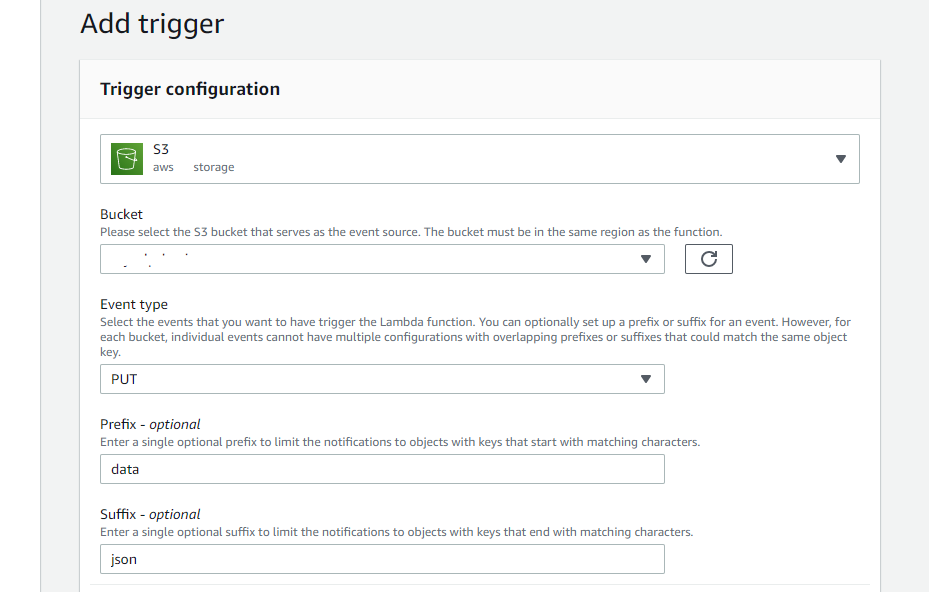# FUNCTION CONFIGURATIONFill in the fields ‘Bucket’, ‘Event type’, ‘Prefix’ and ‘Suffix’ (optional).

# CODE FOR LAMBDA FUNCTION

`const aws = require('aws-sdk);const S3 = new AWS.S3();const Comprehend = new AWS.Comprehend();`
`const params1 = {     Bucket: '<bucket-name>',     Key: 'data/texts.json'}const params2 = {     Bucket: '<bucket-name>',     Key: 'output/results.json',     Body: <object-content>}`
`function getObj() {     return S3.getObject(params1).promise()          .then(data => {               let parsedData = JSON.parse(data.Body.toString());               return parsedData;          })          .catch(err => {               return err;          });}`
`function prcObj(text) {     let res = [];     return Comprehend.detectKeyPhrases({               LanguageCode: 'en',               Text: text          }).promise()          .then(async data => {               for(let i = 0; i < data.KeyPhrases.length; i++) {                    let phrase = await data.KeyPhrases[i].Text;                    res.push(phrase);               }               return res;          })          .catch(err => {               return err;          });}`
`function putObject(body) {     return S3.putObject(params2).promise()          .then(() => {               let msg = 'success! check the 'output' directory.';               return msg;          })          .catch(err => {               return err;          });}`
`exports.handler = event => {     return getObj()          .then(async data => {               console.log('texts: ', data);               let results = [];               for(let i = 0; i < data.length; i++) {                    let phrases = await prcObj(data[i].text);                    results.push(phrases);               }               console.log('results: ', results);               let msg = await putObj(JSON.stringify(results));               return msg;          .catch(err => {               return err;          });}`